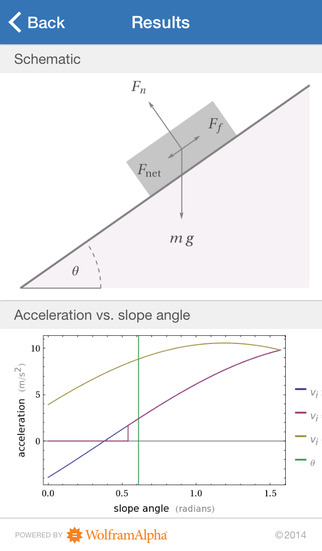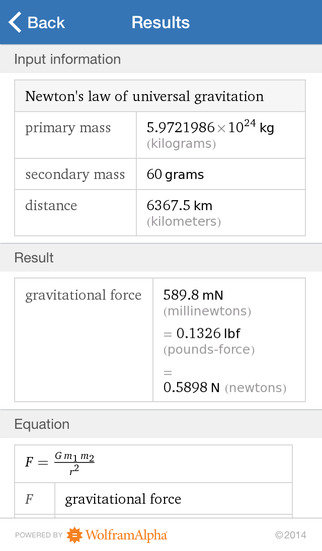5.0

# Wolfram Physics I Course Assistant

• 内容摘要
Taking introductory physics or need a quick physics reference? Then you need the Wolfram Physics I Course Assistant. This app will help you work through your homework problems, ace your tests, and learn physics concepts. Forget canned examples! The Wolfram Physics I Course Assistant solves your specific physics problems on the fly.

This app covers the following topics from Physics I:

- Solve linear and rotational kinematic equations
- Calculate forces of gravity, friction, and springs
- Calculate motion of rockets, pendulums, collisions, and more
- Perform calculations involving mass, weight, density, energy, work, and power
- Do unit conversions
- Look up laws of physics and common physics constants

The Wolfram Physics I Course Assistant is powered by the Wolfram|Alpha computational knowledge engine and is created by Wolfram Research, makers of Mathematica—the world's leading software system for mathematical research and education.

The Wolfram Physics I Course Assistant draws on the computational power of Wolfram|Alpha's supercomputers over a 3G, 4G, or Wi-Fi connection.

展开↓

• 版本中的新功能

展开↓

• 应用截图••#### 同类热门推荐

••iTunes U 3.6 | 56.30MB
•Toca Life: School 1.3 | 225.00MB
•宝宝超市-宝宝巴士 9.12.1000 | 161.00MB
•#### 开发商的其他应用

•WolframAlpha 1.7.3 | 30.40MB
••••#### 不可错过# 安装同步助手后能更便捷的下载此应用• 免费下载游戏和应用
• 快速安装APP
• 便捷管理、优化手机
A# 连接设备至同步助手，自动安装“Wolfram Physics I Course Assistant”

### 安装步骤• 色情内容
• 赌博
• 暴力内容
• 反动内容
• 侵犯版权
• 木马病毒
• 恶意扣费
• 不良插件
• 无法安装或者启动
• 其他